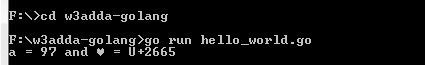# Go Data Types

In this tutorial you will learn about the Go Data Types and its application with practical example.

## Go Data Types

Variables are used to represent reserved memory locations that is used to store values, when we create a variable we are a suppose to allocate some memory space for that variable. Go is a statically typed programming language. This means that variables always have a specific type and that type cannot change. Every variable have data type associated to it, data type for a variable defines –

• The amount of memory space allocated for variables.
• A data type specifies the possible values for variables.
• The operations that can be performed on variables.

Go comes with following built-in data types –

• Numbers
• Boolean
• String

Golang Numbers:- The Number data type is used to hold the numeric values. Go supports following numerical data types –

Integer:- Integers are used to store whole numbers. Go supports several integer types, varying internal sizes for storing signed and unsigned integers.

Signed Integers
Type Size Description Range
int8 8 bits 8 bit Signed Integer (two’s complement) -128 to 127
int16 16 bits 16 bit Signed Integer (two’s complement) -215 to 215 -1
int32 32 bits 32 bit Signed Integer (two’s complement) -231 to 231 -1
int64 64 bits 64 bit Signed Integer (two’s complement). They can also be represented in octal and hexadecimal -263 to 263 -1
int Platform dependent Signed integers of at least 32-bit in size, not equivalent to int32. It is 32 bits wide on a 32-bit system and 64-bits wide on a 64-bit system. Platform dependent
Unsigned Integers
Type Size Description Range
uint8 8 bits 8 bit Unsigned Integer 0 to 127
uint16 16 bits 16 bit Unsigned Integer 0 to 216 -1
uint32 32 bits 32 bit Unsigned Integer 0 to 232 -1
uint64 64 bits 64 bit Unsigned Integer 0 to 264 -1
uint Platform dependent Unsigned integers of at least 32-bit in size, not equivalent to int32. It is 32 bits wide on a 32-bit system and 64-bits wide on a 64-bit system. Platform dependent

Its better to use int data type unless you have a specific reason to use the sized or unsigned integer types. Uninitialized, integral types have a default value of 0. In Golang, octal numbers can be declared using 0 prefix and hexadecimal numbers using the 0x prefix.

Other Integer Types :-

Other Integer Types
Type Description
byte It is alias for and equivalent to uint8
rune It is alias for and equivalent to int32, used to represent characters.
uintptr It is used to hold memory address pointers

Golang do not supports a char data type, instead It have byte and rune to represent character values.This helps to distinguish characters from integer values.

Both byte and rune data types are basically holds integers, the byte data type is represented with a ASCII value while the rune data type is represented with Unicode value encoded in UTF-8 format.

For example, a byte variable with value ‘a’ is converted to the integers its ASCII equivalent 97.Similarly, a rune variable with a unicode value ‘♥’ is converted to the its corresponding unicode equivalent U+2665, here U+ stands for unicode and the numbers are hexadecimal, which is essentially an integer.

Example:-

Output:-Golang Float:- A Float type is used to store numbers that contain a decimal component (real numbers). Go supports float32 and float64 represented with 32-bit and 64-bit in memory respectively. An uninitialized float has default value of 0 and . An untyped floating point values is considered as float64. So in cases of an untyped floating point variable declaration with an initial value without specifying a type explicitly, the compiler will automatically assign float64 type to it.

Example:-

Complex Numbers:- Go supports two additional types for representing complex numbers (numbers with imaginary parts) complex64 and complex128. Each of the type uses float32 and float64 respectively, to represent their real and imaginary parts.

Example:- Complex number can be defined as following –

Here, untyped complex number if inferred as ‘complex128’.

Golang Boolean:- The Boolean data type is used to represent the truth values, which can be either True or False. Boolean are commonly used in decision making statements.

Example:-

Output:-

Golang String:- A string variable is used to hold series or sequence of characters – letters, numbers, and special characters. Strings are immutable. Strings can either be declared using double quotes “Hello World” or back ticks `Hello World`.

Example:-

The difference between these is that double quoted strings cannot contain newlines and they allow escape sequences. For example \n gets replaced with a newline and \t gets replaced with a tab character.

Raw strings are enclosed within back ticks and can span multiple lines, but escape characters don’t have any special meaning to it.

In this tutorial we have learn about the Go Data Types and its application with practical example. I hope you will like this tutorial.